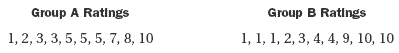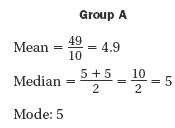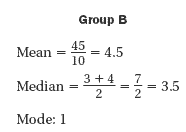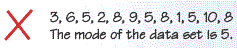Lesson - Mean, Median, Mode, and Range

Mean, Median, Mode, and Range

Three types of averages can be used to describe a data set.

Averages

• The mean of a data set is the sum of the values divided by the number of values.
• The median of a data set is the middle value when the values are written in numerical order. If a data set has an even number of values, the median is the mean of the two middle values.
• The mode of a data set is the value that occurs most often. A data set can have no mode, one mode, or more than one mode.
• The range of a data set is the difference between the largest and smallest values in the set.

Math In Real Life

ExampleA marine biologist records the locations of deep sea jellies in relation to the ocean surface. Jellies are found at -2,278 feet, -1,875 feet, -3,210 feet, -2,755 feet, -2,407 feet, and -2,901 feet.

What is the average location of a deep sea jelly?

Find the Mean

To find the mean of the 6 locations of the deep sea jellies in the problem above, divide the sum of the locations by 6.

The mean location in relation to the ocean surface is -2571 ft.

1) Find the mean of the data.

2) Find the mean of the data.

Finding Median, Mode, and Range

Find the median, mode(s), and range of the movie prices below.

\$7.20, \$13.25, \$14.94, \$16.56, \$18.74, \$19.99, \$19.99, \$29.49

Median

The data set has an even number of prices, so the median is the mean of the two middle values, \$16.56 and \$18.74.

Mode

The price that occurs most often is \$19.99. This is the mode.

Range

The range of a data set is the difference of the greatest value and the least value.

Range = \$29.49-\$7.20=\$22.29

Choosing a Representative Average

Groups A and B try a new ice cream flavor and rate it on a scale of 1 to 10 as shown. Which average best represents each group?SolutionThe mean, median, and mode are very close. So each average is a fair representation of the ratings as a group.The mean is higher than all but 3 ratings. The mode is equal to the lowest rating. So, mean and mode are not good choices. The median best represents the ratings.

3) Find the Error
Describe and correct the error in the solution.4) Use the data set 6, 12, 4, 15, 10, 6, 2, 9 to complete the statement using meanmedianmodeor range

a. The _?_ is 8.

b. The _?_ is 6.

c. The _?_ is 13.

5) Jocelyn is training to run in a race. Her practice times (in minutes and seconds) are 22:45, 21:56, 21:03, 20:33, and 20:28.

Find her mean time to complete the race.

Step 1 Change Jocelyn's practice times to seconds.

Step 2 Find the sum of the practice times. Divide by the number of times.

Step 3 Convert your answer to minutes and seconds.